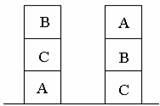Time: 3 Hours                                                                                                     Max. Marks: 100

NOTE: There are 9 Questions in all.

·      Question 1 is compulsory and carries 20 marks.

·      Out of the remaining EIGHT Questions answer any FIVE Questions. Each question carries 16 marks.

·      Any required data not explicitly given, may be suitably assumed and stated.

Q.1       State True or False                                                                                                   (2x10)

a.       Simulated annealing is not a variation of hill climbing.

b.      Operator subgoaling concept is applied in means – ends analysis.

c.       Threshold representing a lower bound on the value that a maximising mode is assigned is called Beta.

d.   Forward chaining is data driven.

e.   Frames provide a way of associating knowledge with objects.

f.    Red cut and Green cuts are concepts of LISP.

g.   Certainty Factor [h, e] = Measure of disbelief [h, e] – Measure of belief       [h, e].

h.   Linear planning is Goal Stack Planning.

i.    Augmented Transition Network does not use bottom up approach.

j.    Dempster shafer theory does not use statistical reasoning.

Answer any FIVE Questions out of EIGHT Questions.

Each question carries 16 marks.

Q.2     a.   Solve the Crypt arithmetic problem using constraint satisfaction and trace the process.                    (8)

CROSS

--------------

DANGER

--------------

b.      Write algorithms and list the advantages & disadvantages of Depth First Search & Breadth First Search.                                                                (8)

Q.3     a.   Explain the types of knowledge representations used in PROLOG with examples.               (8)

b.   Define a predicate in PROLOG to append a list L1 to L2.                                  (4)

c.   Explain how binary tree is represented using PROLOG concepts with examples.                (4)

Q.4     a.   Consider the following block world problem:Solve the problem using linear planning and draw the graph for it.                      (10)

b.   Express the following sentences in First Order Predicate Logic (FOPL) and then convert to clauses.

“All those who honor both their parents are blessed.  If anyone dislikes any of his siblings, one does not honor ones parents.  Mary likes her brother Bill”.                                                              (6)

Q.5     a.   Let C and L be two fuzzy sets of COMFORT and LARGE houses respectively.  If the member grade of a given house H being C is 0.7 and being L is 0.6, then what member grade of the house H is being

(i)                  LARGE and COMFORTABLE

(ii)                LARGE or COMFORTABLE                                                               (4)

b.      Explain and derive Bayes Theorem.                                                                    (4)

c.       Write a resolution Algorithm which can be used for predicate Statements.           (8)

Q.6     a.   Differentiate between Production systems and Non-Production system with example.                     (8)

b.   Explain the different methods of knowledge acquisition.                                       (8)

Q.7           Discuss about the following with examples:-

(i)                  Recursive Transition Network. (8)

(ii)                Frame Systems with merits and demerits.                                                (8)

Q.8     a.   Explain with an example the problem reduction search technique.                        (8)

b.   Explain a simple model of a learning system.  Illustrate through examples the learning by analogy technique.                                                            (8)

Q.9          Write notes on any TWO of the following:-

(i)                  Expert systems and its components

(ii)                Hopfield networks

(iii)               Version Spaces                                                                          (2x8=16)